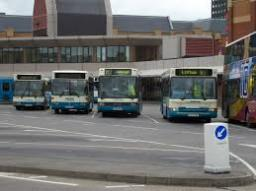# Three-eighths 2783

There were a lot of people standing at the bus stop in the morning. Three-eighths of them got on the first bus, one-fourth on the second, and the remaining 9 on the third. How many people were at the stop from the beginning?

n =  24

### Step-by-step explanation:

n-3/8 · n- 1/4 · n = 9

3n = 72

n = 72/3 = 24

n = 24

Our simple equation calculator calculates it.Did you find an error or inaccuracy? Feel free to write us. Thank you!

Tips for related online calculators
Need help calculating sum, simplifying, or multiplying fractions? Try our fraction calculator.
Do you have a linear equation or system of equations and looking for its solution? Or do you have a quadratic equation?